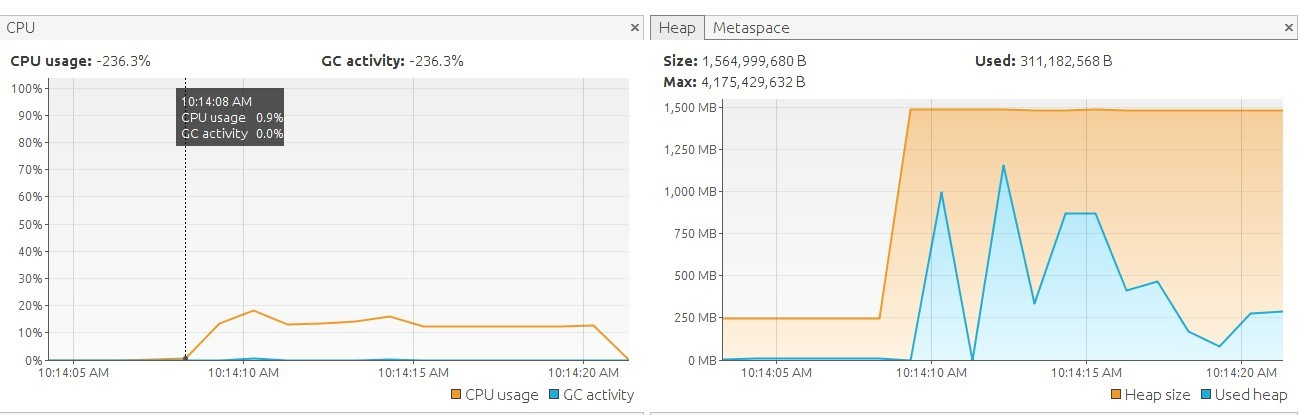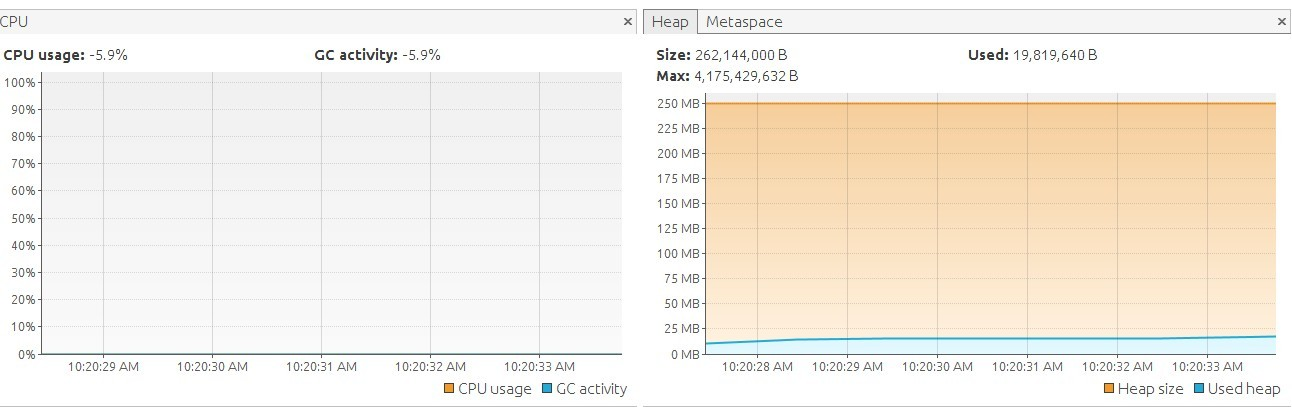# Java 8 Streams - Introduction

You are going to learn :

• basic transformations on Java 8 Streams
• using Streams to perform some more sophisticated queries on data
• various ways of collecting computation results in Sequential Streams
• how Stream differs from other collections and how their Lazy nature may improve performance
• why in Java8 you have Stream and IntStream, why Optional and OptionalInt

Let's Start!

# Part 1 : Transforming Data

At the beginning we will see how to create Streams from classic Java collections and how to transform data in them.

In Java8 all collections have now a default method stream() - method on interface with default implementation which creates a stream.

``````Stream<Integer> stream = Arrays.asList(1, 2, 3, 4, 5).stream()
``````

## Associativity

The main way of transforming data in Stream is a map method (which can be also called high order function).

``````Arrays.asList(1,2,3,4,5).stream()
.map(e->e+1)
.forEach(e->print(e+","))
``````

What is important here to mention is a fact that when we have pure functions we can compose those functions into single map invocation or we can invoke map for each function separately and we will receive the same result. Again - the concept of "pure function" is important here and map method also must obey this rule.

``````Function<String, Integer> parseInt = Integer::parseInt;
Function<Integer,Integer> square=i->i*i;

Function<String, String> composed = parseInt.andThen(square).andThen(e->e+" ");

Stream<String> step1b = Arrays.asList("1", "2", "3", "4", "5").stream();
step1b.map(composed).forEach(this::print);
``````

## Reduction

Other class of operations on Stream transforms the whole Stream into a single value.In a basic form of reduce method the Type of elements and single result must be the same

``````Integer sum = Arrays.asList(1, 2, 3, 4, 5).stream().reduce(0, Integer::sum);
``````

## Exercises

Exercises are split into 3 levels

Level 1

• practice Stream creation and simple mapping

Level 2

• practice map-reduce
• collect elements into an external collection

Level 3

• practice writing generic functions like :
• Generic map-reduce
• Implement map in terms of reduce and forEach

# Part 2 - Data transformation and queries

This time we are going to practice finding answers in a Stream of data. We are going to use some prepared csv file content to simulate searching process.

``````    static String header="ID,FROM,TO,AMOUNT,DATE";
static String line1="1,1,2,100,01-02-2016";
static String line2="2,1,3,200,01-02-2016";
static String line3="3,2,3,50,01-02-2016";
static String line4="4,2,1,666,01-02-2016";
static String line5="5,3,2,500,15-02-2016";
static String line6wrongStructure ="7";
static String line7="6,2,2,100,15-02-2016";
static String line8="7,1,4,100,23-06-2016";
static String line9="8,1,4,100,23-06-2016";
static String line10wrongSyntax ="aaa,bbb,4,200,23-06-2016";
static String line11="9,3,4,700,23-06-2016";
static String line12empty=",,,,";
``````

First step is to prepare data to analysis. Let's remove header :

`````` Transactions.transactions.stream()
.skip(1)
.forEach(this::println);
``````

Yet there are still some malformed lines which you need to filter out - this will be part of our exercises.

# Finding Information

We can use :

• anyMatch & allMatch to analyse whole stream
• findAny & findFirst to finding particular element which fulfills filtering condition
• distinct - for finding unique element
• sorted - for finding elements in particular order
• count,min & max

# flatMap

There will be an occasion to practice usage of this method in exercises. Generally it needs to be used when we want transform each element of a stream with a function which also returns a Stream so we will not end with Type

``````<Stream<Stream<A>>>
``````

For example we need to use it when we want to combine two lists into single list of pairs :

``````        List<Integer> firstList = Arrays.asList(1, 2, 3);
List<Integer> secondList = Arrays.asList(3,4,5);

List<Tuple2<Integer,Integer>> pairs = firstList.stream()
.flatMap(
i -> secondList.stream()
.map(j -> Tuple.of(i,j))
).collect(Collectors.toList());
``````

## Exercises

Level 1

• simple filtering and searching

Level 2

• domain data transformation

Level 3

• filter malformed transactions with flatMap
• implement custom generic filter
• implement custom generic sort

# Part 3 - Collectors

Time to learn how to collect data after transformation.

### Choose collection

Java8 Collectors class provides many ready to use collectors which can save Stream values into given collection type :

• toList()
• toSet()
• toCollection(collectionSupplier)

We can also compose collectors to perform additional steps before returning collection. In the example below we are mapping elements while collecting them into list.

``````List<String> mapped = Stream.of(1, 2, 2, 3).collect(mapping(i -> i.toString()+"str", toList()))
``````

### Join String

This is obvious - comments not needed

``````String withPrefixAndSuffix = Stream.of(1, 2, 3, 4, 5)
.map(i -> i.toString()).collect(joining(",","{","}"));

//with prefix and suffix : {1,2,3,4,5}
``````

### Statistics

While collecting elements we can compute some general statistics which can give us more information about elements in the Stream

``````IntSummaryStatistics statistics = Stream.of("aaa", "bbbb", "ccccccc").collect(Collectors.summarizingInt(String::length));

//IntSummaryStatistics{count=3, sum=14, min=3, average=4.666667, max=7}
``````

### partition and groupBy

Finally we can combine elements into map according to provided criteria

• partition into two groups
• group into multiple groups
`````` Map<NumberSize, List<Integer>> grouped = Stream.of(1, 20000, 3, 400, 5, 6000, 700).collect(groupingBy(i -> {
if (i > 1000) return NumberSize.BIG;
else if (i > 100) return NumberSize.MEDIUM;
else return NumberSize.SMALL;
}));
``````

## Exercises

Level 1

• simple examples of collecting to new collection, grouping and joining

Level 2

• In this part you will practice collectors composition up to 4 different collectors

Level 3

Finally you will write your own Collector!

# Part 4 - Lazy Computation

Let's prepare two functions which will display what is being calculated step by step. Those two functions will help us to illustrate lazy nature of Java Stream.

``````        Predicate<Integer> greaterThanThreeWithLogging = i -> {
println("    * filtering i>3 : " + i);
return i > 3;
};
Function<Integer, Integer> squareWithLogging = i -> {
println("    * mapping i*i : " + i);
return i * i;
};
``````

Now when we run :

``````List<Integer> resultFilterMap = Stream.of(1, 2, 3, 4, 5)
.filter(greaterThanThreeWithLogging)
.map(squareWithLogging).collect(Collectors.toList());
``````

The result is :

``````    * filtering i>3 : 1
* filtering i>3 : 2
* filtering i>3 : 3
* filtering i>3 : 4
* mapping i*i : 4
* filtering i>3 : 5
* mapping i*i : 5
*  calculated result filter map: [16, 25]
``````

So notice that mapping is not executed when filtering removes data value and also that whole chain process value after value without generating intermediate collections!

## Infinity

Laziness allow us to create infinite Streams.

This is infinite stream :

``````Stream.iterate(1, i -> i + 1)
``````

And now we can decide "how much" of infinity we need for our work.

``````List<Integer> firstFiveSquares = Stream
.iterate(1, i -> i + 1)
.map(squareWithLogging)
.limit(5)
.collect(Collectors.toList());
``````

And the result :

``````calculating firts five squares
* mapping i*i : 1
* mapping i*i : 2
* mapping i*i : 3
* mapping i*i : 4
* mapping i*i : 5
* calculated first five squares [1, 4, 9, 16, 25]
``````

## Exercises

There are no levels here just because the nature of infinite streams may be not intuitive for participants so we may want to finish those exercises together.

• take 7 random numbers from infinite stream
• compute sum of first n integers
• compute first n Fibonacci numbers

# Part 5 - Primitives

Till now we worked mainly with boxed types like Integer. But Java8 has separated hierarchy of primitive constructs like IntStream or IntUnaryOperator. What is the purpose of this? Let's check an example

### Boxed

We want to calculate a sum of first 1000000000 numbers. First let's do it with boxing and unboxing.

``````long numberOfElements = 1000000000;

Long result = Stream
.iterate(0L, i -> i + 1)
.map(i->i*i)
.limit(numberOfElements)
.reduce(0L,Long::sum);
``````

And picture below presents what was happening during this operation :We can observe some spikes in memory usage and also CPU was a little bit busy.

### Primitive

Now check a primitive version with special primitive functional types:

`````` LongUnaryOperator step = i -> i + 1;
LongUnaryOperator intSquare = i -> i * i;
LongBinaryOperator sum = (i1, i2) -> i1 + i2;

long primitiveResult = LongStream
.iterate(0, step)
.map(intSquare)
.limit(numberOfElements)
.reduce(0, sum);
``````

And the result :Well actually not much happened...

And also primitive version was about 15 times faster on my machine (i7 4 cores)

``````3338615082255021824
time in : boxed is 13.695 seconds
3338615082255021824
time in : primitives is 1.019 seconds
``````

## From primitive to boxed

We may want to switch between primitive and boxed Streams for various reasons. One reason is that they operate on completely different functional interfaces.

Those are two completely different things :

``````UnaryOperator<Integer> boxedOperator=i->i+1;
IntUnaryOperator primitiveOperator=i->i+1;
``````

Also primitive collectors are less intuitive :

`````` ArrayList<Integer> primitivesCollected = IntStream.of(1, 2, 3, 4, 5, 6, 7, 8, 9)
.map(primitiveOperator)
.collect(ArrayList::new, ArrayList::add, ArrayList::addAll);
``````

And technically in above "collect" we are using there some very exotic types like :

``````@FunctionalInterface
public interface ObjIntConsumer<T> {
void accept(T t, int value);
``````

We can also use switch boxed/unboxed :

``````List<Integer> boxedCollected = IntStream.of(1, 2, 3, 4, 5, 6, 7, 8, 9)
.map(primitiveOperator)
.boxed()
.collect(Collectors.toList());
``````

or move from objects to primitives while transforming the data :

``````nt sumOfTransactions = Stream.of(
Tuple.of("t1", 100),
Tuple.of("t2", 200),
Tuple.of("t3", 300),
Tuple.of("t4", 100)
).mapToInt(t -> t._2)
.sum();
``````

## Exercises

• calculate sum of first n integers - with primitives we don't need reduce method because we have dedicated "int methods"
• find max int in the Stream - here we will see that primitives operate on completely different set of classes like OptionalInt
• Calculate Int sum when on start you have Stream of BigIntegers